# Hydrogen Flow over a Detached V-Shaped Rib in a Rectangular Channel

Hydrogen Flow over a Detached V-Shaped Rib in a Rectangular Channel
Mechanical Engineering Department, Prince Sultan Endowment for Energy and Environment, Prince Mohammad Bin Fahd University, Al-Khobar 31952, Saudi Arabia

Unit of Research on Materials and Renewable Energies, Department of Physics, Faculty of Sciences, Abou Bekr Belkaid University, PB 119-13000-Tlemcen, Algeria

Corresponding Author Email:
achamkha@pmu.edu.sa
Page:
178-186
|
DOI:
https://doi.org/10.18280/mmep.070202
13 May 2019
|
Accepted:
22 July 2019
|
Published:
30 June 2020
| Citation

OPEN ACCESS

Abstract:

In this paper, a new channel model was designed. The study aims to improve the dynamic and thermal behavior of a turbulent flow of hydrogen by introducing a new complex rib between its hot upper and lower walls. The new shape of the rib allows destabilizing flow with the creation of very strong recirculation cells on its back sides, mixing well fluid in the entire interior of the channel, thus a high thermal transfer. The Reynolds averaged Navier-Stokes (RANS) equations, along with the standard k-ε turbulence model and the energy equation, are used to control the channel flow model. All the equations are discretized by the finite volume method by means of a two-dimensional formulation, using the SIMPLE pressure-velocity coupling algorithm. With regard to the flow characteristics, the interpolation QUICK scheme is used, and a second-order upwind scheme is applied for the pressure terms. The results are shown in terms of streamlines, mean and axial velocity fields, dynamic pressure, turbulent kinetic energy, viscosity and temperature fields. This type of analysis is very useful in many industries and engineering related problem for getting good idea about the physical model whenever the analytic solution is out of reach.

Keywords:

V-shaped rib, rectangular channel, turbulent flow, forced convection, hydrogen fluid

1. Introduction

The arrangement of obstacles, such as baffles, fins and ribs, within heat exchange channels are among the effective methods used by many researchers and investigators in their numerical and experimental studies. Liu et al.  conducted an experimental study of heat transfer characteristics in steam-cooled rectangular channels with two opposite rib-roughened walls for Reynolds number (Re) in the range of 10,000 - 80,000. Sethi et al.  carried out an experimental investigation for a range of system and operating parameters in order to analyse the effect of artificial roughness on heat transfer and friction characteristics in solar air heater duct which is having dimple shaped elements arranged in angular fashion (arc) as roughness elements on absorber plate. Eiamsa-ard and Promvonge  numerically investigated the laminar periodic flow and heat transfer characteristics in a channel fitted with triangular wavy baffle elements in tandem, inline arrangements on two opposite walls. Eiamsa-ard et al.  numerically investigated the effects of the baffle at different angles of attack in a channel laminar periodic flow on the flow structure, heat transfer, friction factor and thermal performance behaviors. Jiang et al.  performed numerical simulations for the helical baffles heat exchanger and the segmental baffles heat exchanger with component clearance to reveal the features of leakage streams and their effect on heat exchanger performance. Tan et al.  presented the experimental work carried out to compare the shell-side heat transfer coefficients, Nusselt number, pressure drops and friction coefficients of non-Newtonian nanofluids to those of non-Newtonian base fluid in a helically baffled heat exchanger with low finned tubes for test fluid cooling using water as a coolant. Zhang et al.  provided experimental results for the heat transfer performance and the pressure drop characteristic of the shell sides enhanced by helical fins and vortex generators. Jamshed et al.  performed a detailed CFD analysis on helical grooved tubes with geometry from published articles to validate the results, and three other geometries with variable pitch length aloof from experiment. Zhang et al.  experimentally studied the flow and heat transfer characteristics of various shell-and-tube heat exchangers, one with segmental baffles and four with helical baffles with practical dimensions. Du et al.  analyzed by numerical simulation the influence of various geometric parameters on heat transfer and flow resistance characteristics of overlapped helical baffled heat exchangers. Eiamsa-ard and Promvonge  conducted a numerical prediction to study heat transfer and flow friction behaviors in turbulent channel flows over periodic grooves. Mohsenzadeh et al.  numerically investigated the effect of wall proximity of two isothermal tandem triangle cylinders on fluid flow and heat transfer for different gap spacing in a horizontal plane channel. Ali et al.  reported experimental investigations on steady state forced convection heat transfer from the outer surface of horizontal triangular surface cylinders in cross flow of air. Ozceyhan et al.  presented a numerical study for investigating the heat transfer enhancement in a tube with the circular cross sectional rings. Promvonge et al.  conducted a numerical work to examine turbulent periodic flow and heat transfer characteristics in a three dimensional square-duct with inline 60° V-shaped discrete thin ribs placed on two opposite heated walls. Dutta and Hossain  investigated the local heat transfer characteristics and the associated frictional head loss in a rectangular channel with inclined solid and perforated baffles. Pirouz et al.  carried out a detailed numerical study of the flow and heat transfer through two wall-mounted obstacles placed in lower and upper walls. Wang et al.  reported computational and experimental results for research on the flow and heat transfer process of a rectangular channel embedded with staggered pin fins. Singh et al.  conducted experiments on rectangular duct having one broad wall roughened with discrete V-down rib and subjected to constant heat flux. Skullong et al.  experimentally and numerically investigated the effects of 30° oblique HB inserts at different BR and PR values on turbulent flow and heat transfer behaviors in a square duct. Kumar et al.  carried out an experimental investigation to study the heat transfer and friction characteristics in a solar air heater by using discrete W-shaped roughness. Ary et al.  numerically and experimentally investigated the fluid flow and heat transfer in a rectangular channel with a single inclined baffle and two inclined baffles. Karwa and Maheshwari  presented results of an experimental study of heat transfer and friction in a rectangular section duct with fully perforated baffles (open area ratio of 46.8%) or half perforated baffles (open area ratio of 26%) at relative roughness pitch of 7.2 - 28.8 affixed to one of the broader walls. Mokhtari et al.  investigated the effect of using various fin arrangements on cooling of the base plate in a laminar flow. Mousavi and Hooman  numerically investigated the laminar fluid flow and heat transfer in the entrance region of a two dimensional horizontal channel with isothermal walls and with staggered baffles. Promvonge  experimentally conducted an investigation on heat transfer augmentation in a square duct with insertion of combined 30° V-fins and quadruple counter-twisted tapes. Oztop et al.  reviewed the previously conducted studies and applications in terms of design, performance assessment, heat transfer enhancement techniques, experimental and numerical works, thermal heat storage, effective- ness compassion and recent advances. Alam and Kim  reported a comprehensive literature review of the various heat transfer techniques used to increase the performance of double-pass solar air heaters (SAHs). Kabeel et al.  presented a review of the literature dealing with improvement methods, design configurations and applications of different types of solar air heaters. Other works can be found in the literature [30-40].

Through this study, a new channel model was designed. The study aims to improve the dynamic and thermal behavior of a turbulent flow of hydrogen by introducing a new complex rib between its hot upper and lower walls. The new shape of the rib allows destabilizing flow with the creation of very strong recirculation cells on its back sides, mixing well fluid in the entire interior of the channel, thus a high thermal transfer.

2. Geometry under Investigation

The physical model considered is illustrated in Figure 1. It is a horizontal two-dimensional rectangular channel with hot two upper and lower walls, containing a detached V-shaped rib near its entrance. It is crossed by a flow of hydrogen, its constant properties.

## 1.jpg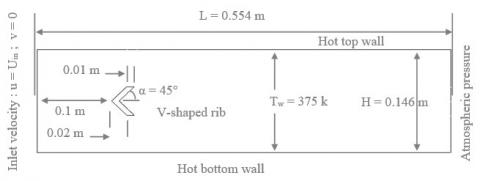Figure 1. Computational domain under investigation

The following assumptions were used to simulate this physical model:

• The flow and heat transfer are two-dimensional.
• The regime is steady and turbulent.
• Physical properties of fluid (hydrogen) and solid (Aluminium) are constant.
• Velocity profile at the inlet is uniform.
• The temperature applied to the upper and lower walls of the channel is constant.
• Radiation heat transfer is negligible.
• The tackiness of the lower and upper walls of the channel is neglected.

Based on these assumptions, the mathematical equations that describe the fluid flow and heat transfer in this channel are as follows:

Continuity equation:

$\frac{\partial}{\partial x_{i}}\left(\rho u_{i}\right)=0$  (1)

Momentum equation:

$\frac{\partial}{\partial x_{j}}\left(\rho u_{i} u_{j}\right)=-\frac{\partial P}{\partial x_{i}}+\frac{\partial}{\rho x_{j}}\left[\mu\left(\frac{\partial u_{i}}{\partial x_{j}}+\frac{\partial u_{j}}{\partial x_{i}}\right)+(-\rho \overline{u_{i}^{\prime} u_{j}^{\prime}})\right]$  (2)

Energy Equation:

$\frac{\partial}{\partial x_{i}}\left(\rho u_{i} T\right)=\frac{\partial}{\partial x_{j}}\left(\left(\Gamma+\Gamma_{t}\right) \frac{\partial T}{\partial x_{j}}\right)$  (3)

where, the second-order tensor of Reynolds stresses $\rho \overline{u_{i}^{\prime} u_{j}^{\prime}}$ is introduced in momentumequation in terms of the Boussinesq hypothesis. The eddy viscosity (μt) defined as

$\mu_{t}=\rho C_{\mu} \frac{k^{2}}{\varepsilon}$  (4)

where, Γ and Γt are molecular thermal diffusivity and turbulent tharmal diffusivity, repectively and are given by

$\Gamma=\mu / \operatorname{Pr} \quad$ and $\quad \Gamma_{t}=\mu_{t} / \operatorname{Pr}_{t}$  (5)

where, ρ is the fluid density, P the pressure, μ dynamic viscosity, ui and uj are average velocity components in x and y directions, respectively.

The standard k-epsilon (ε) model, based on Launder and Spalding , is defined by two transport equations, one for the turbulent kinetic energy, k and the other for the dissipation rate ε, as given below:

$\frac{\partial}{\partial x_{j}}\left(\rho k u_{j}\right)=\frac{\partial}{\partial x_{j}}\left[\left(\mu+\frac{\mu_{t}}{\sigma_{k}}\right) \frac{\partial k}{\partial x_{j}}\right]+G_{k}+\rho \varepsilon$  (6)

$\frac{\partial}{\partial x_{j}}\left(\rho \varepsilon u_{j}\right)=\frac{\partial}{\partial x_{j}}\left[\left(\mu+\frac{\mu_{t}}{\sigma_{\varepsilon}}\right) \frac{\partial \varepsilon}{\partial x_{j}}\right]+C_{1 \varepsilon} \frac{\varepsilon}{k}-C_{2 \varepsilon} \rho \frac{\varepsilon^{2}}{k}$  (7)

In Eqns. (6) and (7), Gk represents the production rate of the kinetic energy due to the energy transfer from the mean flow to turbulence. The empirical constants for the standard k-ε model are assigned the following values :

$\mathrm{C}_{\mu}=0.99 ; \mathrm{C}_{1 \varepsilon}=1.44 ; \mathrm{C}_{2 \varepsilon}=1.92 ; \sigma_{\mathrm{k}}=1.0 ; \sigma_{\varepsilon}=1.3$  (8)

The hydrodynamic boundary conditions are set according to the numerical and experimental analysis of Demartini et al. . The thermal boundary conditions are chosen according to the study of Nasiruddin and Siddiqui .

At the channel intake:

$u(0, y)=U_{i n}$  (9a)

$v(0, y)=0$  (9b)

$T(0, y)=T_{i n}=300 k$  (9c)

The kinetic energy of turbulence (k) and its dissipation rate (ε) are defined, respectively, as

$k(0, y)=k_{i n}=0,005 . U_{i n}^{2}$  (10a)

$\varepsilon(0, y)=\varepsilon_{i n}=0,1 \cdot k_{i n}^{2}$  (10b)

At the upper and lower walls of the channel:

$u=v=0$  (11a)

$k=\varepsilon=0$  (11b)

$T=T_{w}=375 k$  (11c)

Additionally, the points of solid-fluid contact must maintain continuity of both temperature and heat flux such that

$T_{s}=T_{f}$  (12a)

And

$k_{s} \frac{\partial T_{s}}{\partial n}=k_{f} \frac{\partial T_{f}}{\partial n}$  (12b)

where, the partial derivative with respect to n indicates a normal derivative, kf and ks are thermal conductivities of fluid and solid, respectively.

At the channel:

$P(L, y)=P_{a t n}$  (13a)

$\frac{\partial \phi}{\partial x}(L, y)=0$  (13b)

where, ϕ ≡ (u, v, T, k, ε). The flow Reynolds number Re, based on channel hydraulic diameter Dh,

$D_{h}=2 H W /(H+W)$  (14a)

is given by:

$\operatorname{Re}=\rho U_{i n} D_{h} / \mu$  (14b)

3. Approach

The governing flow equations, i.e., continuity, momentum, and energy, are solved numerically by finite volume method  using the commercial CFD software FLUENT. The SIMPLE (Semi Implicit Method for Pressure Linked Equations) algorithm  is used for pressure velocity coupling. The QUICK (Quadratic Upstream Interpolation for Convective Kinetics) numerical scheme  is selected to discretize the convective terms in the governing equations. The SOU (Second-Order Upwind) numerical scheme  is used for the pressure terms. The Standard k-epsilon (ε) model  is used to describe the turbulence. A two-dimensional non-uniform grid is used. Figure 2 illustrates the refined mesh at all solid boundaries.

## 2.jpg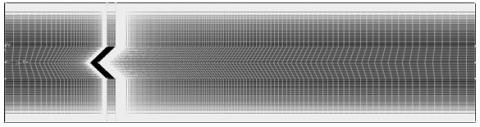Figure 2. Mesh

The grid independence test was performed by comparing various sets of the grid cells (95 × 35), (120 × 45), (145 × 55), (170 × 65), (195 × 75), (220 × 85), (245 × 95) and (370 × 145) on the umax for Re = 8.73 × 104. The grid cell, with (245 × 95) nodes along the X and Y directions respectively, showed about 0.150 per cent deviation from the values umax, as compared to those of the grid cell (370 × 145). Therefore, the grid with (245 × 95) nodes is chosen for all cases.

## 3.jpgFigure 3. Verification of f0 for smooth channel

The present numerical results of friction factor (f0) for smooth channel with no rib are compared with the results obtained from correlations of Petukhov and Blasius . This comparison is shown in Figure 3. It is very clear that there is a good quantitative agreement between them and this proves the validity of our simulation.

4. Results and Discussion

Figure 4 a, b, c, d and e shows the contour plots of dynamic pressure fields (P) for hydrogen flow in the case of five different values of Reynolds number, i.e., Re = 5.000, 10.000, 15.000, 20.000 and 25.000, respectively.

## 4.jpgFigure 4. Fields of dynamic pressure (P) for (a) Re = 5.000; (b) Re = 10.000; (c) Re = 15.000; (d) Re = 20.000; (e) Re = 25.000. P values in Pa

## 5.pngFigure 5. Pmax values for various Re values

It is very clear that the pressure values are very high on the upper and lower edges of the v-rib, across the gaps, next to the top and bottom surfaces of the channel. P values decrease on the front side of the rib, while they are very low on the back side between its wings, and on its front sharp edge. As expected, P values improve with Reynolds values and Re = 25.000 indicates the pest pression value. This was also confirmed in Figure 5. There is a direct correlation between Reynolds numbers and pressure values due to the increase in fluid velocity at the input, where pressure increases through the gaps due to the presence of the v-rib.

## 6.jpg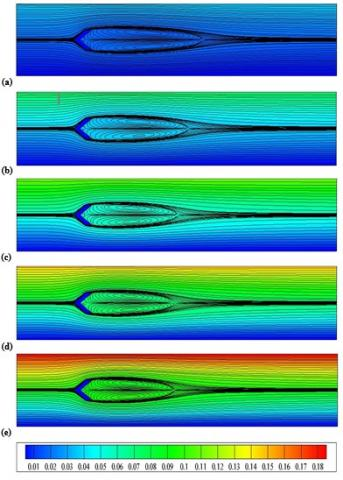Figure 6. Fields of stream function (Ψ) for (a) Re = 5.000; (b) Re = 10.000; (c) Re = 15.000; (d) Re = 20.000; (e) Re = 25.000. Ψ values in kg/s

## 7.png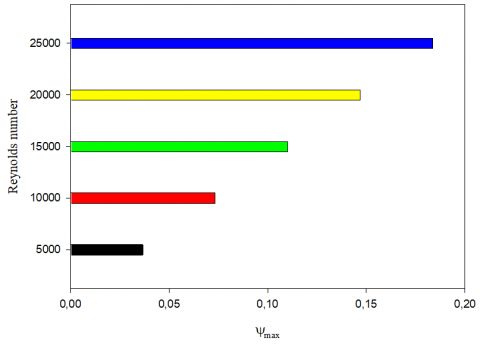Figure 7. Ψmax values for various Re values

The contour plots of stram function fields (Ψ) are shown in Figure 6 (a-e) for various Re values. The hydrogen current lines are very turbulent near the v-rib, while they are regular and parallel near the upper and lower walls of the channel. The current has constant speed at the channel intake with regular lines. The current is disturbed as it approaches the front of the v-rib. The current passes through the top and bottom gaps, near the upper and lower sharp edges of the v-rib, along parallel lines to the exit of the channel, while these lines are disturbed on the right side of the same obstacle, where two recycling cells are formed next to its upper and lower wings due to low pressure in this region. As also shown in Figure 6, the strength of these zones of recirculation increases with increasing Re values. Ψ values are important for the large Re values, as shown in Figure 7.

## 8.jpg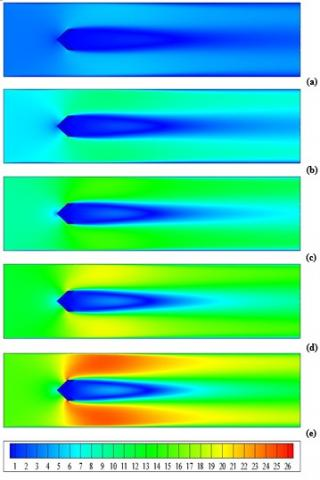Figure 8. Fields of mean velocity (V) for (a) Re = 5.000; (b) Re = 10.000; (c) Re = 15.000; (d) Re = 20.000; (e) Re = 25.000. V values in m/s

Figure 8 shows the variation of the mean velocity (V) with the Re value. The V values decreases after the channel entrance on the front region of the v-rib. The presence of this obstacle in the middle of the channel and near its intake allows the division and direction of the hydrogen current at very high speeds towards the gaps that are located near the upper and lower sharp edges of the same obstacle. This increase in velocity is due to an increase in pressure values across these narrow areas due to the presence of the v-rib. The top and bottom front wings of the v-rib changes the flow direction and creates a large disturbance near its upper and lower tips. At the back of this obstacle, the V values are very low as a result of the presence of a large recycling zone extending from the right sides of its upper and lower wings to the middle of the channel. The V values are very high near the hot surfaces of the channel in the regions opposite the upper and lower wings, especially for the large Revalues, Figures 8 and 9. This increase in speed decreases as the flow approaches the channel outlet, Figures 8.

## 9.png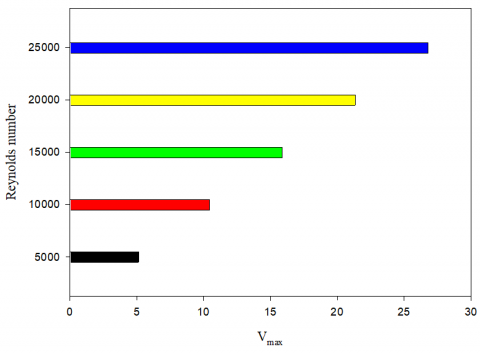Figure 9. Vmax values for various Re values

## 10.jpgFigure 10. Fields of axial velocity (u) for (a) Re = 5.000; (b) Re = 10.000; (c) Re = 15.000; (d) Re = 20.000; (e) Re = 25.000. V values in m/s

The distribution of axial velocity fields (u) in the ribbed channel for five various Re values is presented in Figure 10. As shown in the figure, there are negative values for velocity. These weak values are present on the back region of the v-rib, which extend from its inside to the middle of the channel. These negative values indicate that there are recycling cells in this region. These cells are reverse currents that flow in the opposite direction. In the regions between the upper and lower edges and the channel walls, the u values are very high. As expected, the change in Re values has a significant impact on the flow velocity, Figures 10 and 11. The u value augments with the augmentation of Re number and thus, the Re = 25.000 provides maximum axial velocity, Figures 10 and 11. Therefore, there is an increase in u values with Re numbers (see Figures 10 and 11) where the intensity, strength, size and extension of recirculation zones improve by improving Re values, Figures 6 and 7.

## 11.pngFigure 11. umax values for various Re values

## 12.jpg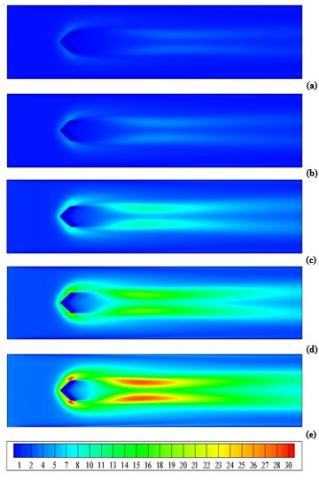Figure 12. Fields of turbulent kinetic energy (k) (a) Re = 5.000; (b) Re = 10.000; (c) Re = 15.000; (d) Re = 20.000; (e) Re = 25.000. k values in m2/s2

The contour plots of turbulence kinetic energy fields are shown in Figure 12 for various values of Re number. The turbulence kinetic energy values are very high next to the front sharp edges of the top and bottom sides of the v-rib as well as on its back side at the right of the recirculation zone to the outlet of the channel. As is very clear, the turbulence kinetic energy is greatly increased by increasing the Re value where reaches its maximum value (kmax) in the case of Re = 25.000 as shown in Figure 12, and as confirmed by Figure 13.

Figure 14 shows the simulated data of turbulent viscosity using the k-epsilon (k-ε) model of turbulence in the range of Re number examined. The values of turbulent viscosity are considered on all areas of the channel except for the fluid surfaces near the top and bottom walls of the channel as well as next to the right side of the v-rib. The turbulent viscosity reaches its highest values on the upper and lower wings in its front. As indicated, there is a significant increase in turbulent viscosity values during the rise in Re number values, Figures 14 and 15.

## 13.png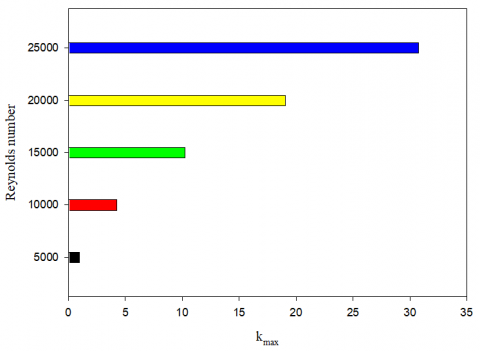Figure 13. kmax values for various Re values

## 14.jpg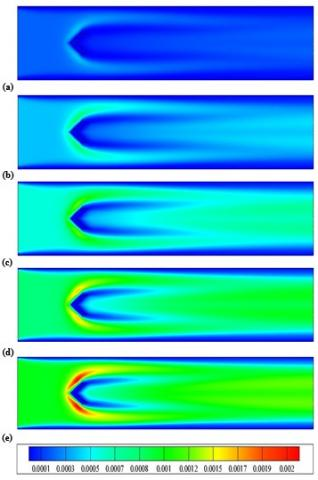Figure 14. Fields of turbulent viscosity (μt) for (a) Re = 5.000; (b) Re = 10.000; (c) Re = 15.000; (d) Re = 20.000; (e) Re = 25.000. μt values in kg/m-s

The distribution of fluid temperature in a v-ribbed channel for five various Re values is addressed in Figure 16. It is very clear that the presence of the v-rib in the center of the channel near its entrance allows the current to be directed towards the hot upper and lower walls of the channel and thus acquire more thermal energy. The temperature gradient enhances next to the v-rib. These regions have good heat transfer. While the temperature gradient decreases as the flow approaches the channel exit. As expected, the heat transfer between the fluid and the hot surfaces is important for the large values of Re number.

## 15.png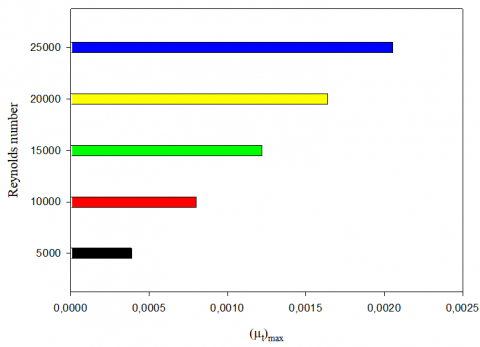Figure 15.t)max values for various Re values

## 16.jpgFigure 16. Fields of temperature (T) for (a) Re = 5.000; (b) Re = 10.000; (c) Re = 15.000; (d) Re = 20.000; (e) Re = 25.000. T values in k

5. Conclusion

The most important conclusions that can be drawn from this study are as follows:

- The pressure (P) values are very high on the upper and lower edges of the v-rib, across the gaps, next to the top and bottom surfaces of the channel. P values decrease on the front side of the rib, while they are very low on the back side between its wings, and on its front sharp edge.

- The current passes through the top and bottom gaps, near the upper and lower sharp edges of the v-rib, along parallel lines to the exit of the channel, while these lines are disturbed on the right side of the same obstacle, where two recycling cells are formed next to its upper and lower wings due to low pressure in this region.

- The top and bottom front wings of the v-rib changes the flow direction and creates a large disturbance near its upper and lower tips. At the back of the v-rib, the velocity values are very low as a result of the presence of a large recycling zone extending from the right sides of its upper and lower wings to the middle of the channel. These cells are reverse currents that flow in the opposite direction.

- The turbulence kinetic energy values are very high next to the front sharp edges of the top and bottom sides of the v-rib as well as on its back side at the right of the recirculation zone to the outlet of the channel. The presence of the v-rib in the center of the channel near its entrance allows the current to be directed towards the hot upper and lower walls of the channel and thus acquire more thermal energy. As indicated above, there is a significant increase in pressure, velocity, turbulent kinetic energy and turbulent viscosity values during the rise in Re number values. The intensity, strength, size and extension of recirculation zones improve by improving Re values.

- This type of analysis is very useful in many industries and engineering related problem for getting good idea about the physical model whenever the analytic solution is out of reach.

References

 Liu, J.Z., Gao, J.M., Gao, T.Y., Shi, X.J. (2013). Heat transfer characteristics in steam-cooled rectangular channels with two opposite rib-roughened walls. Applied Thermal Engineering, 50(1): 104-111. https://doi.org/10.1016/j.applthermaleng.2012.05.003

 Sethi, M., Varun, Thakur, N.S. (2012). Correlations for solar air heater duct with dimpled shape roughness elements on absorber plate. Solar Energy, 86(9): 2852-2861. https://doi.org/10.1016/j.solener.2012.06.024

 Eiamsa-ard, S., Promvonge, P. (2012). Laminar periodic flow and heat transfer in a rectangular channel with triangular wavy baffles. Journal of Thermal Science, 21: 250-261. https://doi.org/10.1007/s11630-012-0542-5

 Eiamsa-ard, S., Pattanapipat, S., Promvonge, P. (2013) Influence of triangular wavy baffles on heat and fluid flow characteristics in a channel. Journal of Mechanical Science and Technology, 27: 2199-2208. https://doi.org/10.1007/s12206-013-0534-8

 Jiang, B., Yan, S.H., Zhang, L., Xiao, X. (2017). Numerical research of stream analysis on helical baffles heat exchangers. Journal of Engineering Thermophysics, 26: 272-290. https://doi.org/10.1134/S1810232817020102

 Tan, Y.K., He, Z.B., Xu, T., Fang, X.M., Gao, X.N., Zhang, Z.G. (2017). Experimental investigation of heat transfer and pressure drop characteristics of non‑Newtonian nanofluids flowing in the shell-side of a helical baffle heat exchanger with low‑finned tubes. Heat Mass Transfer, 53: 2813-2827. https://doi.org/10.1007/s00231-017-2015-6

 Zhang, L., Guo, H., Wu, J., Du, W. (2012). Compound heat transfer enhancement for shell side of double-pipe heat exchanger by helical fins and vortex generators. Heat Mass Transfer, 48: 1113-1124. https://doi.org/10.1007/s00231-011-0959-5

 Jamshed, S., Qureshi1, S.R., Shah, A., Hussain, A. (2018). Investigation of entropy generation rate and its minimization in helical grooved tubes. Journal of Mechanical Science and Technology, 32(10): 4983-4991. https://doi.org/10.1007/s12206-018-0946-6

 Zhang, J.F., Li, B., Huang, W.J., Lei, Y.G., He, Y.L., Tao, W.Q. (2009). Experimental performance comparison of shell-side heat transfer for shell-and-tube heat exchangers with middle-overlapped helical baffles and segmental baffles. Chemical Engineering Science, 64(8): 1643-1653. https://doi.org/10.1016/j.ces.2008.12.018

 Du, T., Du, W., Che, K., Cheng, L. (2015). Parametric optimization of overlapped helical baffled heat exchangers by Taguchi method. Applied Thermal Engineering, 85: 334-339. https://doi.org/10.1016/j.applthermaleng.2015.02.058

 Eiamsa-ard, S., Promvonge, P. (2008). Numerical study on heat transfer of turbulent channel flow over periodic grooves. International Communications in Heat and Mass Transfer, 35(7): 844-852. https://doi.org/10.1016/j.icheatmasstransfer.2008.03.008

 Mohsenzadeh, A., Farhadi, M., Sedighi, K. (2010). Convective cooling of tandem heated triangular cylinders confirm in a channel. Thermal Science, 14(1): 183-197. https://doi.org/10.2298/TSCI1001183M

 Ali, M., Zeitoun, O., Nuhait, A. (2011). Forced convection heat transfer over horizontal triangular cylinder in cross flow. International Journal of Thermal Sciences, 50(1): 106-114. https://doi.org/10.1016/j.ijthermalsci.2010.09.007

 Ozceyhan, V., Gunes, S., Buyukalaca, O., Altuntop, N. (2008). Heat transfer enhancement in a tube using circular cross sectional rings separated from wall. Applied Energy, 85: 988-1001. https://doi.org/10.1016/j.apenergy.2008.02.007

 Promvonge, P., Changcharoen, W., Kwankaomeng, S., Thianpong, C. (2011). Numerical heat transfer study of turbulent square-duct flow through inline V-shaped discrete ribs. International Communications in Heat and Mass Transfer, 38(10): 1392-1399. https://doi.org/10.1016/j.icheatmasstransfer.2011.07.014

 Dutta, P., Hossain, A. (2005). Internal cooling augmentation in rectangular channel using two inclined baffles. International Journal of Heat and Fluid Flow, 26(2): 223-232. https://doi.org/10.1016/j.ijheatfluidflow.2004.08.001

 Pirouz, M.M., Farhadi, M., Sedighi, K., Nemati, H., Fattahi, E. (2011). Lattice Boltzmann simulation of conjugate heat transfer in a rectangular channel with wall-mounted obstacles. Scientia Iranica B, 18(2): 213-221. https://doi.org/10.1016/j.scient.2011.03.016

 Wang, F. M., Zhang, J., Wang, S. (2012). Investigation on flow and heat transfer characteristics in rectangular channel with drop-shaped pin fins. Propulsion and Power Research, 1(1): 64-70. https://doi.org/10.1016/j.jppr.2012.10.003

 Singh, S., Chander, S., Saini, J.S. (2011). Heat transfer and friction factor correlations of solar air heater ducts artificially roughened with discrete V-down ribs. Energy, 36: 5053-5064. https://doi.org/10.1016/j.energy.2011.05.052

 Skullong, S., Thianpong, C., Jayranaiwachira, N., Promvonge, P. (2016). Experimental and numerical heat transfer investigation in turbulent square-duct flow through oblique horseshoe baffles. Chemical Engineering and Processing, 99: 58-71. https://doi.org/10.1016/j.cep.2015.11.008

 Kumar, A., Bhagoria, J.L., Sarviya, R.M. (2009). Heat transfer and friction correlations for artificially roughened solar air heater duct with discrete W-shaped ribs. Energy Conversion and Management, 50(8): 2106-2117. https://doi.org/10.1016/j.enconman.2009.01.025

 Ary, B.K.P., Lee, M.S., Ahn, S.W., Lee, D.H. (2012). The effect of the inclined perforated baffle on heat transfer and flow patterns in the channel. International Communications in Heat and Mass Transfer, 39(10): 1578-1583. https://doi.org/10.1016/j.icheatmasstransfer.2012.10.010

 Karwa, R., Maheshwari, B.K. (2009). Heat transfer and friction in an asymmetrically heated rectangular duct with half and fully perforated baffles at different pitches, International Communications in Heat and Mass Transfer, 36(3): 264-268. https://doi.org/10.1016/j.icheatmasstransfer.2008.11.005

 Mokhtari, M., Gerdroodbary, M.B., Yeganeh, R., Fallah, K. (2017). Numerical study of mixed convection heat transfer of various fin arrangements in a horizontal channel. Engineering Science and Technology, an International Journal, 20(3): 1106-1114. https://doi.org/10.1016/j.jestch.2016.12.007

 Mousavi, S.S., Hooman, K. (2006). Heat and fluid flow in entrance region of a channel with staggered baffles. Energy Conversion and Management, 47: 2011-2019. https://doi.org/10.1016/j.enconman.2005.12.018

 Promvonge, P. (2015). Thermal performance in square-duct heat exchanger with quadruple V-finned twisted tapes. Applied Thermal Engineering, 91: 298-307. https://doi.org/10.1016/j.applthermaleng.2015.08.047

 Oztop, H.F., Bayrak, F., Hepbasli, A. (2013). Energetic and exergetic aspects of solar air heating (solar collector) systems. Renewable and Sustainable Energy Reviews, 21: 59-83. https://doi.org/10.1016/j.rser.2012.12.019

 Alam, T., Kim, M.H. (2017). Performance improvement of double-pass solar air heater - A state of art of review. Renewable and Sustainable Energy Reviews, 79: 779-793. https://doi.org/10.1016/j.rser.2017.05.087

 Kabeel, A.E., Hamed, M.H., Omara, Z.M., Kandeal, A.W. (2017). Solar air heaters: Design configurations, improvement methods and applications - a detailed review. Renewable and Sustainable Energy Reviews, 70: 1189-1206. https://doi.org/10.1016/j.rser.2016.12.021

 Hajipour, M., Dehkordi, A.M. (2014). Mixed-convection flow of Al2O3-H2O nanofluid in a channel partially filled with porous metal foam: experimental and numerical study. Experimental Thermal and Fluid Science, 53: 49-56. https://doi.org/10.1016/j.expthermflusci.2013.11.002

 Zeeshan, A., Shehzad, N., Ellahi, R., Alamri, S.Z. (2018). Convective Poiseuille flow of Al2O3-EG nanofluid in a porous wavy channel with thermal radiation. Neural Computing and Application, 30: 3371-3382. https://doi.org/10.1007/s00521-017-2924-9

 Hayat, T., Rani, S., Alsaedi, A., Rafiq, M. (2017). Radiative peristaltic flow of magneto nanofluid in a porous channel with thermal radiation. Results in Physics, 7: 3396-3407. https://doi.org/10.1016/j.rinp.2017.07.074

 Hosseini, S.R., Ghasemian, M., Sheikholeslami, M., Shafee, A., Li, Z. (2019). Entropy analysis of nanofluid convection in a heated porous microchannel under MHD field considering solid heat generation. Powder Technology, 344: 914-925. https://doi.org/10.1016/j.powtec.2018.12.078

 Hussain, S., Mehmood, K., Sagheer, M., Farooq, A. (2017). Entropy generation analysis of mixed convective flow in an inclined channel with cavity with Al2O3-water nanofluid in porous medium. International Communications in Heat and Mass Transfer, 89: 198-210. https://doi.org/10.1016/j.icheatmasstransfer.2017.10.009

 Pourmehran, O., Rahimi-Gorji, M., Hatami, M., Sahebi, S.A.R., Domairry, G. (2015). Numerical optimization of microchannel heat sink (MCHS) performance cooled by KKL based nanofluids in saturated porous medium. Journal of the Taiwan Institute of Chemical Engineers, 55: 49-68. https://doi.org/10.1016/j.jtice.2015.04.016

 Job, V.M., Gunakala, S.R. (2018). Unsteady hydromagnetic mixed convection nanofluid flows through an L-shaped channel with a porous inner layer and heat-generating components. International Journal of Heat and Mass Transfer, 120: 970-986. https://doi.org/10.1016/j.ijheatmasstransfer.2017.12.112

 Kothandapani, M., Prakash, J. (2014). Effect of radiation and magnetic field on peristaltic transport of nanofluids through a porous space in a tapered asymmetric channel, Journal of Magnetism and Magnetic Materials, 378: 152-163. https://doi.org/10.1016/j.jmmm.2014.11.031

 Maghrebi, M.J., Nazari, M., Armaghani, T. (2012). Forced convection heat transfer of nanofluids in a porous channel. Transport Porous Media, 93: 401-413. https://doi.org/10.1007/s11242-012-9959-2

 Matin, M.H., Pop, I. (2013). Forced convection heat and mass transfer flow of a nanofluid through a porous channel with a first order chemical reaction on the wall. International Communications in Heat and Mass Transfer, 46: 134-141. https://doi.org/10.1016/j.icheatmasstransfer.2013.05.001

 Mohyud-Din, S.T., Jan, S.U., Khan, U., Ahmed, N. (2016). MHD flow of radiative micropolar nanofluid in a porous channel: optimal and numerical solutions. Neural Computing and Application, 29: 793-801. https://doi.org/10.1007/s00521-016-2493-3

 Launder, B.E., Spalding, D.B. (1974). The numerical computation of turbulent flow. Computer Methods in Applied Mechanics and Engineering, 3(2): 269-289. https://doi.org/10.1016/0045-7825(74)90029-2

 Demartini, L.C., Vielmo, H.A., Möller, S.V. (2004). Numeric and experimental analysis of the turbulent flow through a channel with baffle plates. Journal of the Brazilian Society of Mechanical Sciences and Engineering, 26(2): 153-159. https://doi.org/10.1590/S1678-58782004000200006

 Nasiruddin, M.K., Siddiqui, K. (2007). Heat transfer augmentation in a heat exchanger tube using a baffle, International Journal of Heat and Fluid Flow, 28(2): 318-328. https://doi.org/10.1016/j.ijheatfluidflow.2006.03.020

 Patankar, S.V. (1980). Numerical Heat Transfer and Fluid Flow. Hemisphere, New York, USA.

 Leonard, B.P., Mokhtari, S. (1990). Ultra-sharp nonoscillatory convection schemes for High-Speed steady multidimensional flow. NASA TM1-2568, NASA Lewis Research Center.

 Incropera, F.P., Witt, P.D., Bergman, T.L., Lavine, A.S. (2006). Fundamentals of Heat and Mass Transfer. John-Wiley & Sons.